# Search

About 178 Search Results Matching Types of Worksheet, Worksheet Section, Generator, Generator Section, Grades matching 4th Grade, Similar to Valentine's Day Addition Worksheet, Page 4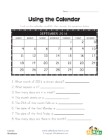## Using a Calendar Worksheet

Look at the calendar and then answer the questions...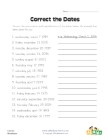## Correct the Dates Worksheet

Correct the punctuation and capitalization of the ...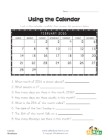## Using a Calendar Worksheet

Look at the calendar and then answer the questions...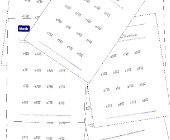## Simple Division Worksheets

This group of worksheets makes a good introduction...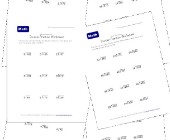## Division Practice Worksheets

This collection of division practice worksheets is...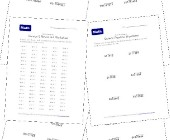## Long Division Worksheets

Help kids practice long division with this set of ...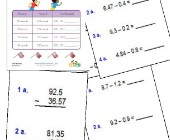## Subtracting Decimals Worksheets

These worksheets will help children practice decim...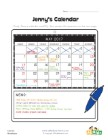## Reading a Calendar Worksheet

Answer the questions using the calendar as a refer...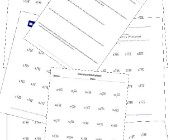## Division Worksheets

Help kids learn division with this collection of m...